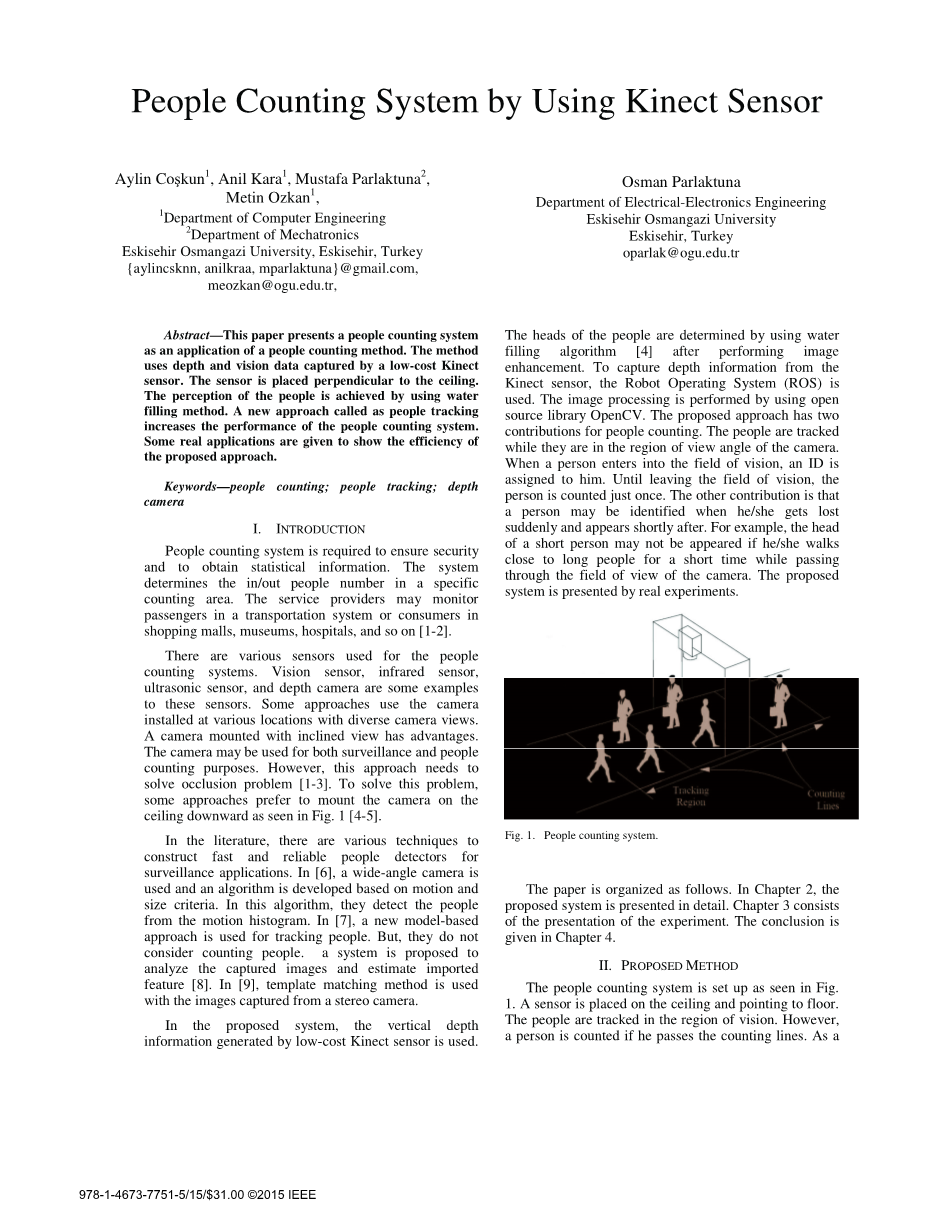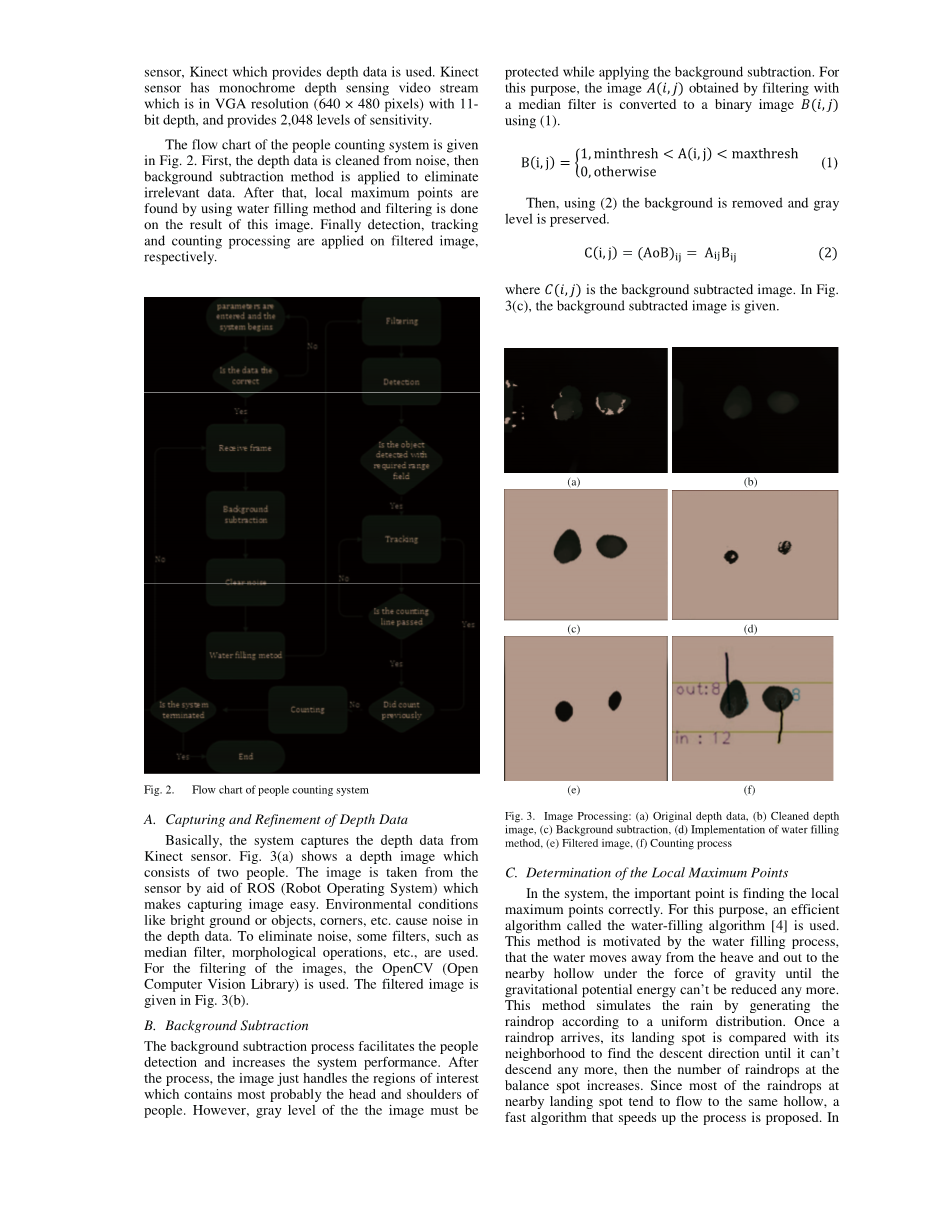# 基于kinect的人数计数系统外文翻译资料

2021-11-08 10:11# Aylin Co kun1、Anil Kara1、穆斯塔法Parlaktuna2、Metin Ozkan1、

Eskisehir Osmangazi大学，埃斯基谢希尔，土耳其

# 奥斯曼Parlaktuna

1. 介绍

1. 提出的方法

（机器人操作系统）从传感器获取图像，这使得捕获图像变得容易。明亮的地面或物体，角落等环境条件会在深度数据中产生噪音。为了消除噪声，使用一些滤波器，例如中值滤波器，形态学运算等。对于图像的过滤， 使用OpenCV （ 开放计算机视觉库）。滤波后的图像如图3（b）所示。

(b)

(c) (d)

(e) (f)

(a)

(b)

(c)

(d)

（b）雨滴的持续时间，（c）从层中流出的雨滴，

（d）注水的结果

KalmanFilter：估计下一个点序列的坐标。

g（x，y）的形式可以是微不足道的，例如零函数。我们期望使用g（x，y）来推断f（x，y）。适当的函数g（x，y）可以具有以下三个优点：

（x，y）。函数f（x，y）的形式可以看作是具有隆起和凹陷的土地。驼峰中的雨滴将在重力作用下直接流向邻域。一点一点地，空心区域会聚集很多雨滴。函数g（x， y）反映了（x，y）处的雨滴数量。雨停后，降雨量大的地区可归类为空心。

1输入:

0, 大小M*N,阈值T, 雨滴数量K.

2: for k=1:K do

3: x = rand(1, M), y = rand(1, N)

4: while True do

5: d(xn, yn) = f(xn, yn) g(yn, yn)

minus;

(f(x, y)

g(x, y)), where (xn, yn) is the neighborhood of

(x, y).

6: (x , y ) = arg min d(xn, yn)

7: if d(x , y ) lt; 0 then

8: x = x , y = y ;

9: else

10: g(x, y) = g(x, y) 1;

break;

11: end if

12: end while

13: end for

14: 使用T对g（x，y）进行阈值处理，然后使用轮廓

15: 输出: 轮廓线.

1：输入：深度图像: f(x, y) and 测量函数 g(x, y) =

0, 大小M*N,阈值T, 雨滴数量K.

2：for k =1: K do

3: x = rand(1, M), y = rand(1, N), w = R

4: while w gt; 0 do

5: d(xn, yn) = f(xn, yn) g(yn, yn)

minus;

(f(x, y)

g(x, y)), where (xn, yn) is the neighborhood of

(x, y).

6: (x , y ) = arg min d(xn, yn)

7: if d(x , y ) r lt;